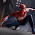## Search This Blog

THE BEAUTY OF MATHEMATICS- Collected from The Internet and Various Books to enrich The students and Teachers. SUPPORT with YOUR COMMENTS ...

## Monday, June 14, 2010

### PERMUTATIONS AND COMBINATIONS

In common, students who come across the topic “Permutation and Combination” feel it to be confusing.

The basic idea of the topic is selection and arrangement of things. They are widely used in solving problems of probability, genetic engineering and life sciences.

Combination to unlock a safe.. Consider a 3 digit number 653 which you use as a combination to the safe. Here the order of the number is important as 563 will not work to unlock the safe. This is permutation where the order of elements is very important.

Combination of three colors.. Consider 4 colors red, blue, yellow and green. If I ask you to select 3 colors and you choosing them as Red, green and blue is same when you choose it as blue, red and green. This is combination where the order does not matter.

To make it simple, mathematically it can be stated as:

“Permutation is an ordered Combination”.

An easy way to memorize:
Permutation  - Position important

Combination - Chosen important
Permutation:
Permutation is arrangement of values in all possible ways.The word arrangement is used to emphasize that the order of the things is important.
Consider the three letter word “PEN”. In how many ways can we rearrange it.
PEN   PNE   EPN   ENP   NEP   NPE
A three letter word can be arranged in 6 ways. You can easily find it out. How about a 10 letter word. Quite a long process isn’t it. Hence we use FACTORIAL to find the number of possible ways to arrange the elements.
For a 3 letter word,

3! = 3*2*1 = 6 ways.

For a 10 letter word,
10! = 10*9*8*7*6*5*4*3*2*1= 3628800
Permutation with Repetition:

Number of permutations of n-things, taken ‘r’ at a time when each thing can be repeated r-times is given by = n ^ r.

A child has 3 pocket and 4 coins. In how many ways can he put the coins in his pocket.
First coin can be put in 3 ways, similarly second, third and forth coins also can be put in 3 ways.

So total number of ways = 3 x 3 x 3 x 3
= 3 ^ 4 = 81

Permutation without Repetition:

If we have to select 3 numbers from a set of 9 numbers say, from 1 to 9 , then what are the possibilities?

For the first number we have 9 choices{1,2,3,4,5,6,7,8,9}. Let us choose 4.

For the second number we have 8 choices{1,2,3,5,6,7,8,9}. Let us choose 8.

For the third number we have 7 choices{1,2,3,5,6,7,9}. Let us choose 3.

We had 9 choices at first, then 8 and then 7.Therefore the total no. of options would be 9*8*7 = 504.

So, if you wanted to select all of the 9 numbers, the permutation would be:

9! =9*8*7*6*5*4*3*2*1 = 362880.

But here you wanted to select just 3, then you have to stop the multiplying after 7. How do you do that? There is a simple trick ... you divide by 6! ...

9! ÷6! = (9*8*7*6*5*4*3*2*1) / (6*5*4*3*2*1)
= 9*8*7 = 504.

Mathematically, the number of permutations of ‘r ‘objects chosen from a set of ‘n’ objects is expressed as:
nPr = n! / (n-r)!

Combination:

Combination means selection of things. The word selection is used, when the order of things has no importance.

If 9 players are selected to form a team from 20 players, the order in which the 9 players are selected doesn’t matter as they are all in the team. This is combination. There will be a change in combination iff a player in the team is changed.

In permutation the order is important as 234 is different from 324. but in combination we are concerned only with the numbers 2, 3 and 4 that have been selected. The combination of 234 is same as 324.

The combinations of abcd taken 3 at a time :

abc abd acd bcd.

Each of these four combinations will give rise to 3! Permutations:
abc     abd     acd     bcd

bca      bda     cda     cdb

cab      dab     dac     dbc

cba     dba     dca     dcb

Each column is the permutation of that combination. But they are all one combination as order has no importance.Since the order does not matter in combinations, there are clearly fewer combinations than permutations. Hence combinations can be stated as :

"Combinations are subsets of permutation".

Thus if we want to figure out how many combinations we have of n objects then r at a time, we just create all the permutation and then divide by r! variant.

Mathematically, the number of combinations of n things taken r at a time is:
nCr = nPr / r!

= n! / [r! (n- r)!]

nCr is known as n choose r. It is also known as Binomial co-efficient.

1.thanks for explaining in a very simple manner..
can you please explain the last part

how r! convert to {r!( n-r) }

thanks

2.hi medha. It is by substituting the value of nPr in nCr . i.e., nCr = nPr / r!
= [n!/(n-r)!](1/r!)
= n! / [r! (n- r)!]

3.nice explanation

4.amazing article...never thought PnC could be this easy..thank you so much

5.awesome explanation with a simple and lucid manner..

6.7.hmmmmm helpd me a lot

8.good explanation.thanks a lot

9.thanks for good exolanation

10.thanks a lot for such a nice explanation

11.simply awesome....!!!!

12.Very interesting, informative & helpful article.
Thank you. :-)

13.the artical is real fascinating to read!

14.